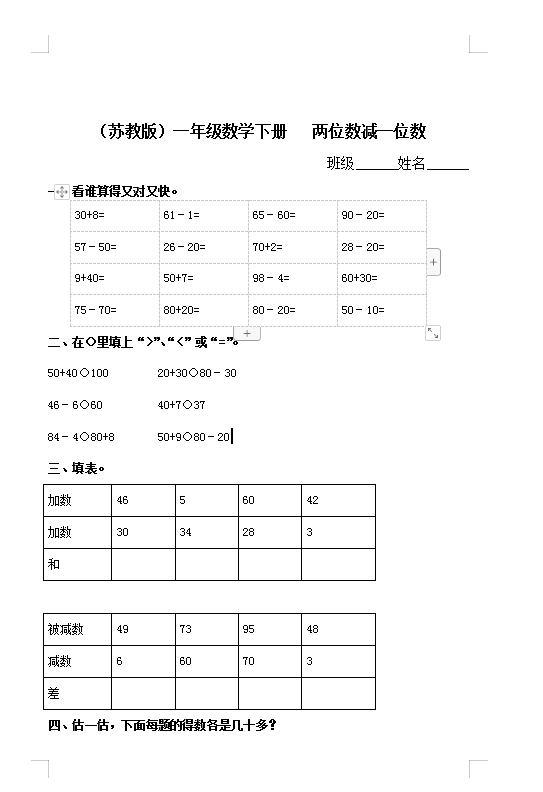（苏教版）小学一年级数学下册 两位数减一位数及答案 2（4页DOC文档）资料下载

（苏教版）小学一年级数学下册   两位数减一位数

 30+8= 61－1= 65－60= 90－20= 57－50= 26－20= 70+2= 28－20= 9+40= 50+7= 98－4= 60+30= 75－70= 80+20= 80－20= 50－10=